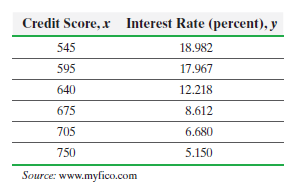×
Get Full Access to Statistics: Informed Decisions Using Data - 5 Edition - Chapter 14.1 - Problem 12
Get Full Access to Statistics: Informed Decisions Using Data - 5 Edition - Chapter 14.1 - Problem 12

×

# ?Credit Scores An economist wants to determine the relation between one’s FICO score, x, and the interest rate of a 36-month auto loan, y. The data repISBN: 9780134133539 240

## Solution for problem 12 Chapter 14.1

Statistics: Informed Decisions Using Data | 5th Edition

• Textbook Solutions
• 2901 Step-by-step solutions solved by professors and subject experts
• Get 24/7 help from StudySoup virtual teaching assistantsStatistics: Informed Decisions Using Data | 5th Edition

4 5 1 293 Reviews
30
0
Problem 12

Credit Scores An economist wants to determine the relation between one’s FICO score, x, and the interest rate of a 36-month auto loan, y. The data represent the interest rate (in percent) a bank might offer on a 36-month auto loan for various FICO scores.Use the results from Problem 18 in Section 4.2 to answer the following questions:(a) Treating credit score as the explanatory variable, x, determine the estimates of0 and1.

(b) Compute the standard error of the estimate, Se.

(c) Determine Sb1.(d) A normal probability plot of the residuals indicates it is reasonable to conclude the residuals are normally distributed. Test whether a linear relation exists between credit score and interest rate at the= 0.05 level of significance.

(e) Construct a 95% confidence interval about the slope of the true least-squares regression line.

Step-by-Step Solution:

Step 1 of 5) Credit Scores An economist wants to determine the relation between one’s FICO score, x, and the interest rate of a 36-month auto loan, y. The data represent the interest rate (in percent) a bank might offer on a 36-month auto loan for various FICO scores. Use the results from Problem 18 in Section 4.2 to answer the following questions: (a) Treating credit score as the explanatory variable, x, determine the estimates of 0 and 1. (b) Compute the standard error of the estimate, Se. (c) Determine Sb1. (d) A normal probability plot of the residuals indicates it is reasonable to conclude the residuals are normally distributed. Test whether a linear relation exists between credit score and interest rate at the = 0.05 level of significance. (e) Construct a 95% confidence interval about the slope of the true least-squares regression line.

Step 2 of 2

## Discover and learn what students are asking

Statistics: Informed Decisions Using Data : Inference about Two Population Standard Deviations
?In Problems 9–14, assume that the populations are normally distributed. Test the given hypothesis. 10. s1 ? s2 at the a = 0.1 level of signif

Unlock Textbook Solution